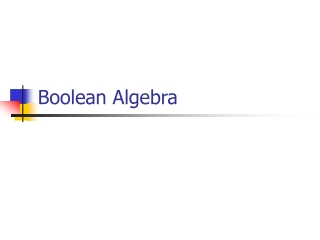DownloadDownload PresentationBoolean Algebra

# Boolean Algebra

Télécharger la présentation## Boolean Algebra

- - - - - - - - - - - - - - - - - - - - - - - - - - - E N D - - - - - - - - - - - - - - - - - - - - - - - - - - -
##### Presentation Transcript

1. Boolean Algebra

2. LOGIC GATES • Boolean variable: Takes only two values – either true (1) or false (0). • Boolean function: Mapping from Boolean variables to a Boolean value. • Truth table: • Represents relationship between a Boolean function and its binary variables. • It enumerates all possible combinations of arguments and the corresponding function values.

3. Continue… • Boolean algebra: Deals with binary variables and logic operations operating on those variables. • Logic diagram: Composed of graphic symbols for logic gates. A simple circuit sketch that represents inputs and outputs of Boolean functions.

4. Gates • Refer to the hardware to implement Boolean operators. • The most basic gates are: • Inverter • OR • AND • XOR • NAND • NOR

5. Continue…

6. Continue…

7. BASIC IDENTITIES OF BOOLEAN ALGEBRA • Postulate 1(Definition): A Boolean algebra is a closed algebraic system containing a set K of two or more elements and the two operators · and + which refer to logical AND and logical OR

8. Basic Identities of Boolean Algebra(Existence of 1 and 0 element) • x + 0 = x • x · 0 = 0 • x + 1 = 1 • x · 1 = x (Table 1-1)

9. Basic Identities of Boolean Algebra (Existence of complement) (5) x + x = x (6) x · x = x (7) x + x’ =1 (8) x · x’ = 0

10. Basic Identities of Boolean Algebra (Commutativity): (9) x + y = y + x (10) xy = yx

11. Basic Identities of Boolean Algebra (Associativity): (11) x + ( y + z ) = ( x + y ) + z (12) x (yz) = (xy) z

12. Basic Identities of Boolean Algebra (Distributivity): (13) x ( y + z ) = xy + xz (14) x + yz = ( x + y )( x + z)

13. Basic Identities of Boolean Algebra (DeMorgan’s Theorem) (15) ( x + y )’ = x’ y’ (16) ( xy )’ = x’ + y’

14. Basic Identities of Boolean Algebra (Involution) (17) (x’)’ = x

15. Function Minimization using Boolean Algebra Examples: (a) a + ab = a(1+b)=a (b) a(a + b) = a.a +ab=a+ab=a(1+b)=a (c) a + a'b = (a + a')(a + b)=1(a + b) =a+b (d) a(a' + b) = a. a' +ab=0+ab=ab

16. Try • F = abc + abc’ + a’c

17. The other type of question Show that; 1- ab + ab' = a 2- (a + b)(a + b') = a

18. More Examples • Show that; (a) ab + ab'c = ab + ac (b) (a + b)(a + b' + c) = ac + bc (a) ab + ab'c = a(b + b'c) = a((b+b').(b+c))=a(b+c)=ab+ac (b) (a + b)(a + b' + c) = (a.a + a.b' + a.c + ab +b.b' +bc) = …

19. DeMorgan's Theorem • F = ab + c’d’ • F’ = ?? • F = ab + c’d’ + b’d • F’ = ??

20. DeMorgan's Theorem Show that: (a + b.c)' = a'.b' + a'.c'

21. More Examples (a(b + c) + a'b)'=b'(a' + c') ab + a'c + bc = ab + a'c (a + b)(a' + c)(b + c) = (a + b)(a' + c)